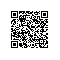# 简单实用的c++快速排序模板类

（一）目标

（二）快速排序算法的思想

1 分解(Divide)：将输入的序列L[p..r]划分成两个非空子序列L[p..q]和L[q+1..r]， 使L[p..q]中任一元素的值不大于L[q+1..r]中任一元素的值。
2 递归求解(Conquer)：通过递归调用快速排序算法分别对L[p..q]和L[q+1..r]进行排序。
3 合并(Merge)：由于对分解出的两个子序列的排序是就地进行的， 所以在L[p..q]和L[q+1..r]都排好序后不需要执行任何计算L[p..r]就已排好序。
（三）准备工作和源代码
1 使用vc6建立console工程
2 加入下面的模板类：

template<typename DataType>//DataType是模板参数，代表了欲排序的数据类型 　　class QuickSortTemp  　　{ 　　public: 　　 QuickSortTemp() 　　 { 　　 } 　　  　　 ~QuickSortTemp() 　　 { 　　 } 　　  　　public: 　　 // 快速排序的实现，Array是要排序数据的数组，nLower，nUpper范围是0 ~ 数据总个数-1 　　 static void QuickSort(DataType* Array, int nLower, int nUpper) 　　 { 　　 // 测试是否排序完毕 　　 if (nLower < nUpper) 　　 { 　　 // 分解和分别进行排序 　　 int nSplit = Partition (Array, nLower, nUpper);//数据切分为两个部分 　　 QuickSort (Array, nLower, nSplit - 1);//左半部分递归排序 　　 QuickSort (Array, nSplit + 1, nUpper);//右半部分递归排序 　　 } 　　 }

// 切分数据为左右两个部分，返回中间元素x的编号
// 主要的过程就是：选择一个元素x作为分界点，将比x大的元素放到x右边，其余放到x左边。

 static int Partition (DataType* Array, int nLower, int nUpper) 　　 { 　　 int nLeft = nLower + 1; 　　 DataType Pivot = Array[nLower]; 　　  　　 int nRight = nUpper; 　　  　　 DataType Swap; 　　 while (nLeft <= nRight) 　　 { 　　 while (nLeft <= nRight && Array[nLeft].CompareTo(Pivot) <= 0) 　　 nLeft = nLeft + 1; 　　 while (nLeft <= nRight && Array[nRight].CompareTo(Pivot) > 0) 　　 nRight = nRight - 1; 　　 if (nLeft < nRight) 　　 { 　　 Swap = Array[nLeft]; 　　 Array[nLeft] = Array[nRight]; 　　 Array[nRight] = Swap; 　　 nLeft = nLeft + 1; 　　 nRight = nRight - 1; 　　 } 　　 } 　　  　　 Swap = Array[nLower]; 　　 Array[nLower] = Array[nRight]; 　　 Array[nRight] = Swap; 　　 return nRight; 　　 } 　　};

以上就实现了快速排序的模板类。
3 数据类接口的实现
从上面模板类的实现我们可以看出，为了使用这个模板类对某种类型的数据数组DataType * data进行排序，我们必须实现DataType的接口CompareTo（比较两个DataType 元素a,b的大小，a>b返回1，a==b返回0，否则返回-1）。
举个例子来说：现在要排序二维点坐标，定义大小关系是：先比较x轴坐标值大小，x相同的话，由y值大小决定大小关系。即：(1,1) == (1,1) , (2,1) > (1, 10) , (3, 5) < (4, 1)。
此外：还必须实现DataType类型的无参数的默认构造函数（因为模板类中要使用）。
定义数据类型MyPoint如下：

struct MyPoint 　　{ 　　 MyPoint() 　　 { 　　 } 　　  　　 MyPoint(int x, int y) 　　 { 　　 this->x = x; 　　 this->y = y; 　　 } 　　 int CompareTo(MyPoint& b) 　　 { 　　 if(this->x < b.x) 　　 return -1; 　　 else if(this->x > b.x) 　　 return 1; 　　 else 　　 { 　　 if(this->y > b.y) 　　 return 1; 　　 else if(this->y < b.y) 　　 return -1; 　　 else 　　 return 0; 　　 } 　　 } 　　 int x; 　　 int y; 　　};

（四）测试
下面是用于测试的主函数：

int _tmain(int argc, TCHAR* argv[], TCHAR* envp[]) 　　{ 　　 int nRetCode = 0; 　　 //Point数组 　　 MyPoint points = {MyPoint(1,1), MyPoint(2,5), MyPoint(7,11), MyPoint(100,2), 　　 MyPoint(1, 7), MyPoint(9,32), MyPoint(7, 1), MyPoint(2,2),  　　 MyPoint(1,1), MyPoint(9,5)}; 　　 int count = 10; 　　 //排序前 　　 printf("before quicksort:/n"); 　　 for(int i = 0 ; i <count ; i ++) 　　 printf("%d <-------> (%d,%d)/n", i, points[i].x, points[i].y); 　　  　　 //调用模板类排序 　　 QuickSortTemp<MyPoint>::QuickSort(points, 0, count - 1); 　　 //排序后 　　 printf("after quicksort:/n"); 　　 for(i = 0 ; i <count ; i ++) 　　 printf("%d <-------> (%d,%d)/n", i, points[i].x, points[i].y); 　　 system("pause"); 　　 return nRetCode; 　　}

结果输出如下:
before quicksort:
0 <-------> (1,1)
1 <-------> (2,5)
2 <-------> (7,11)
3 <-------> (100,2)
4 <-------> (1,7)
5 <-------> (9,32)
6 <-------> (7,1)
7 <-------> (2,2)
8 <-------> (1,1)
9 <-------> (9,5)
after quicksort:
0 <-------> (1,1)
1 <-------> (1,1)
2 <-------> (1,7)
3 <-------> (2,2)
4 <-------> (2,5)
5 <-------> (7,1)
6 <-------> (7,11)
7 <-------> (9,5)
8 <-------> (9,32)
9 <-------> (100,2)
请按任意键继续 . . .
（五）说明

本文根据快速排序算法，实现了一个c++快速排序模板类。使用这个模板类，并遵守欲排序数据类型必须实现的接口定义，就能实现对任意数据类型的快速排序。当然，本文的例子只是一个基本的引导。使用钉钉扫一扫加入圈子
+ 订阅